# State tomography via weak measurements

## Abstract

Recent work has revealed that the wave function of a pure state can be measured directly and that complementary knowledge of a quantum system can be obtained simultaneously by weak measurements. However, the original scheme applies only to pure states and it is not efficient because most of the data are discarded by post-selection. Here, we propose tomography schemes for pure states and for mixed states via weak measurements and our schemes are more efficient because we do not discard any data. Furthermore, we demonstrate that any matrix element of a general state can be directly read from an appropriate weak measurement. The density matrix (with all of its elements) represents all that is directly accessible from a general measurement.

## Introduction

For a group of blindfolded observers, who can each only touch one part of the elephant, to construct an accurate representation of an elephant, the group must combine information about different parts of the elephant (FIG 1a). In quantum mechanics, to have a complete description of a quantum system, in particular, a complete knowledge of a quantum state, we must combine information about complementary aspects (FIG 1b).

An ideal (strong) quantum measurement of a certain observable only gives the probabilities of obtaining the eigenvalues of the observable and the statistical results of the measurements reflect the diagonal terms of the density matrix in the eigenbasis of the observable. Measurements of different (complementary) observables provide the diagonal terms of the density state for different bases. If we perform measurements for a sufficient number of bases, we can reconstruct the density state, which is the idea of state tomography.

An important difference exists between the tomography of a quantum state and its classical counterpart, e.g., the description of an elephant by blindfolded observers. In this classical example, the description (of the elephant) by each man is not exclusive and the different aspects of perception can be simply combined to produce an overall description of the elephant (FIG 1a). However, quantum physics forbids simultaneous knowledge of complementary observables because precise knowledge of a certain aspect necessarily implies uncertainty for the complementary aspects1. Therefore, one cannot simultaneously perform ideal measurements of complementary observables in quantum physics (FIG 2a). To determine the quantum state of a system, different measurement setups, each for a particular observable, are required. To determine the general state of a d-dimensional quantum system, at least d + 1 different experimental setups are needed. Each setup must be devoted to the measurement of one of the complementary observables.

An alternative state tomography approach is possible. Instead of obtaining maximum information of a particular observable by an ideal measurement, we can perform a weak measurement2. The state of the quantum system is only slightly changed during its weak interaction with the measuring device; therefore, weak measurements of a set of complementary observables can be performed simultaneously3 (FIG 2b). Thus, fewer experimental setups are needed for state tomography via weak measurements4.

Weak measurements have been well described in the literature. Since they were first introduced by Aharonov, Albert and Vaidman2, weak measurements have been realised in experiments5,6,7 and have provided new insights into the study of paradoxes and fundamental problems in quantum theory8,9,10,11,12. Weak measurement has also been used as a practical tool for amplifying weak signals and signal-to-noise ratios13,14,15,16,17,18,19,20. Signal amplification via weak measurement usually involves a pre-selection and a post-selection that are nearly orthogonal and the original formalism of weak measurement2,21 is not sufficient for the phenomena in this regime because it only retains the first-order terms of the interaction strength22,23,24. Extensions to the case of general pre-selection are given in (Refs. 25, 26). A framework that retains the high-order terms for general pre-selection and post-selection is given in (Ref. 27) and specific cases are further studied in (Refs. 28, 29, 30). A nonperturbative theory of weak pre- and post-selected measurements is given in (Ref. 31). The general results for the weak measurement of a pair of complementary observables are provided in (Ref. 20). Related concepts, such as the contextual values32 and modular values33, were also introduced. Weak measurements can also be used for state tomography. Lundeen et al.4 directly measured the wave function of a pure state by a weak measurement followed by a post-selection. The scheme was extended to the mixed state by sequential weak measurements of pairs or triple products of complementary observables34. The results of the weak measurements could also be interpreted in terms of the Kirkwood-Dirac representation via complex joint probabilities35,36. Reviews and additional references on weak measurements can be found in (Refs. 31, 37, 38).

The results in (Ref. 4) demonstrate that the wave function of a pure state can be directly obtained from a single experimental setup and that one can directly measure both the absolute values and the phases of the coefficients of a pure state in a certain basis. However, the scheme proposed in4 applies only to pure states and it is not efficient because most of the data are discarded due to the post-selection. Here, we propose tomography schemes for both pure states and mixed states via weak measurements. Our schemes are more efficient because we do not discard any data. We also show that any (diagonal or off-diagonal) element of the density state can be directly determined as the average pointer shift in an appropriate weak measurement.

## Results

### The idea

In the scheme proposed in (Ref. 4), the wave function to be measured is a continuous function of the position. The essence of the scheme is reviewed in finite dimensional Hilbert space as follows34,39. Suppose a quantum system with a d-dimensional Hilbert space is in an unknown state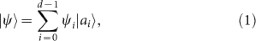where the coefficients {ψi} in a certain basis {|ai〉} need to be determined. The scheme for measuring the coefficients {ψi} consists of a series of weak measurements of the observables Ai = |ai〉 〈ai|, each followed by the same postselection, i.e., a projection onto the final state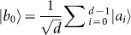. The weak value of the observable Ai is defined as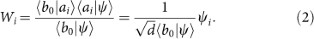Both the real and the imaginary parts of the weak value Wi have physical meanings and can be determined experimentally because they correspond to the average shifts of the pointer position and the momentum, respectively. From Eq. (2), we know that the weak values Wi are directly proportional to the coefficients ψi that we want to measure and that the factorcan be determined by the normalisation condition (up to an unphysical overall phase of |ψ〉). Therefore, we obtain a direct measurement of the coefficients ψi, thus a direct measurement of the wave function |ψ〉.

The essential point in this scheme is the choice of the post-selected state |b0〉. Its overlap with each basis state |ai〉 has the same magnitude and phase; therefore, the factor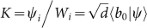does not depend on i and can be determined by normalisation. Because the change of the system state due to a weak interaction with the pointer is negligible, the success probability of post-selection is given by P = |〈b0|ψ〉|2. Therefore, only a fraction P of the data is retained and the remainder is discarded due to the post-selection. When the dimension d is large, as in the case of a continuous wave function, the majority of the data is discarded.

### State tomography of a pure state

The part of the data that corresponds to the failure of the post-selection can be retained. We replace the final post-selection by a complete projective measurement onto the basis states {|bj〉}. The inner products βji = 〈bj|ai〉 are fixed when the two sets of the basis states are chosen. We organise the data according to the final state: if the final state is |bj〉, then the weak value of Ai = |ai〉 〈ai| is given by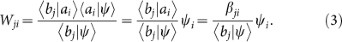Therefore, for each fixed j, the weak values of different Ai give the relative ratios of the coefficients ψi: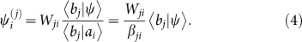Because 〈bj|ψ〉 does not depend on i, the coefficients ψi are directly proportional to, where the weak values Wji are read-outs directly from the experiments and the constants βji = 〈bj|ai〉 are fixed when the two sets of basis states are chosen. The super index (j) indenotes the coefficients ψi that we obtain from the jth subset of the data. Ideally,should not depend on j; however, they may depend on j due to imperfections and noise in the experiments. The magnitude of the factor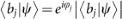can be removed by normalisation: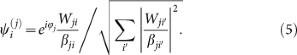The phase factor ϕj has no physical meaning because it corresponds to the overall phase of the pure state we are attempting to measure. This factor can be removed by a convention. For example, we require ψ0 > 0 (or the coefficient with the largest magnitude be positive). Using this approach, we retain all of the data and separate the data into d subsets according to the final states {|bj〉}. For the jth subset of the data that corresponds to the fixed final states |bj〉, we have an estimation of the coefficientsfrom Eq. (4) or (5). A different subset of the data gives a different estimation for the set of coefficients {ψi}. The differences between the estimations indicate the amount of error or noise in the experiment.

When the basis {|bj〉} is mutually unbiased to the basis {|ai〉}, i.e.,, (5) can be simplified to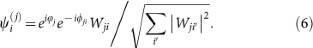The original scheme proposed in4 corresponds to the case in whichand only the data corresponding to the successful post-selection of |b0〉 are retained. Here, we retain all of the data and each subset of the weak values corresponding to a fixed final state gives an estimation of the state to be measured. The probability of obtaining the final state |bj〉 is given by |〈bj|ψ〉|2 because the change of the system state during the weak interaction is negligible. When the state |ψ〉 to be measured is almost orthogonal to a certain final state |bj〉, then the relative frequency to obtain the final state |bj〉 in the post-selection is small. However, the corresponding weak value has a large magnitude, which could result in a large signal-to-noise ratio when the dominant noise is due to systematic error or imperfections.

In the above scheme, weak measurements for a complete set of projective operators {Ai = |ai〉〈ai|} are needed. For the tomography of a pure-state wave function |ψ〉, weak measurements of a single observable instead of a set of observables are sufficient. This single observable can be chosen as follows (an alternative choice is presented in the Methods). We consider a pure state |ϕ〉 such that its overlap with each postselected state is nonzero, i.e., 〈bj|ϕ〉 ≠ 0 for each j. We perform a weak measurement of Pϕ = |ϕ〉〈ϕ|. When the post-selected state is |bj〉, the weak value of Pϕ is defined as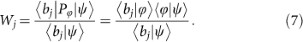Therefore,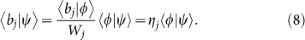Here,is completely determined from the experimental data and our choice because Wj is directly obtained from the experiment and both |ϕ〉 and {|bj〉} are fixed by our choice. From Eq. (8), we can reconstruct the state |ψ〉: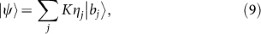where K is determined by the normalisation condition up to a phase.

### State tomography of a mixed state

To this point, we have only considered tomography schemes for a pure state. If the state we like to measure is a general mixed state ρ, we also have a tomography scheme via weak measurements. For this case, we perform weak measurements for a complete set of projective operators Ai = |ai〉〈ai|, followed by a final projective measurement onto the basis {|bj〉}. For the initial state ρ and the final post-selected state |bj〉, the average shifts of the pointer position and the momentum in a weak measurement of Ai correspond to the real and imaginary parts of the weak value25,27From Eq. (10), one can express the matrix elements of ρ either by the basis {|ai〉}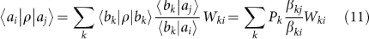or by the basis {|bj〉}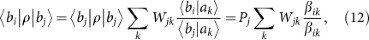where the probability Pj = 〈bj|ρ|bj〉 corresponds to the frequency of obtaining the final state |bj〉 in the experiment. The weak values Wji and the probabilities Pj are directly accessible from the experiment. The relationships in Eqs. (11) and (12) show that the matrix elements of the mixed state can be written as linear summations of the weak values that are directly accessible from the experiment.

### Partial state tomography

Using the idea of weak measurements, we can also selectively measure some of the matrix elements (diagonal and off-diagonal terms) of a state directly. This is especially useful when we are only interested in one or a few matrix elements of the state and we do not need to perform state tomography for the entire density matrix. Suppose we are interested in measuring a particular matrix element 〈a|ρ|b〉. We consider two different cases according to whether |a〉 and |b〉 are orthogonal. For the first case, suppose 〈b|a〉 ≠ 0. Here, we can simply perform a weak measurement of |a〉〈a|, with a post-selection onto the state |b〉. The weak value is defined as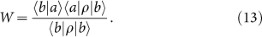Thus,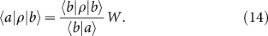W is the weak value and 〈b|ρ|b〉 is the probability of success for post-selection. Both values are directly obtained from the experiment. 〈b|a〉 is only a fixed factor. Therefore, 〈a|ρ|b〉 is determined by Eq. (14). For the second case, suppose that 〈b|a〉 = 0. We then perform a weak measurement of the observable C = |c〉 〈c| withand a follow-up projective measurement onto a basis including both |a〉 and |b〉 as the basis states. We must only retain the data when the final state is either |a〉 or |b〉. If the final state is |a〉, then the weak value of C is given byIf the final state is |b〉, then the weak value of C is given by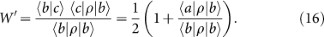We have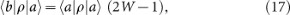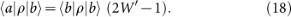The post-selection probabilities 〈a|ρ|a〉 and 〈b|ρ|b〉 and the weak values W and W′ are directly accessible from the experiment; therefore, we can determine the matrix element 〈a|ρ|b〉 from either Eq. (17) or (18), which should agree with each other. Any discrepancies between them could be used as indicators of the noise and imperfections in the experiment.

### Experimental scheme

Here, we discuss how our schemes can be realised experimentally. For the weak measurement of an observable A, the interaction between the measuring device and the system is generally modelled by a Hamiltonian, where p denotes the momentum of the pointer (measuring device). Because the interaction in a weak measurement does not change the state of the system significantly, weak measurements of several observables (whether they are commutative or not) can be performed simultaneously. The interaction of the simultaneous weak measurements of a set of observables {Ai} can be introduced by the Hamiltonian: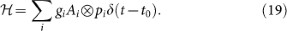Here, pi denotes the momentum of the ith pointer that is coupled to the observable Ai. {Ai} is an arbitrary set of observables of the system. They could be a set of commuting observables (for example, Ai = |ai〉 〈ai|) or even a set of complementary observables. Suppose the initial state of the system is ρs and the initial state of the pointers is···, where ρi denotes the initial state of the ith pointer. Suppose the post-selection of the system is a projection(for example,= |bj〉 〈bj|). Then the average position shift δqi and the average momentum shift δpi of the ith pointer are given by (see the derivation in the Methods)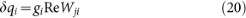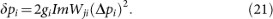The weak value Wji of Ai, for a general initial state ρs and a general postselection, is given by20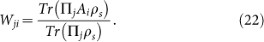The shifts of different pointers can be read out simultaneously, while either the position shift or the momentum shift is recorded at each time for each pointer. With the same Hamiltonian, we can obtain all of the weak values Wji. From these weak values, we can obtain all of the elements of the density matrix ρs according to the state tomography strategy proposed in this article.

### Example of application

The state tomography schemes proposed here can also be used to detect a tiny parameter encoded in a quantum state. For example, to detect a tiny phase difference ϑ picked up by two orthogonal states of a qubit (a photon) that passes through a certain medium (a birefringent crystal), we could prepare a qubit in the initial stateand seek to determine the tiny phase ϑ encoded in the outgoing state. To determine ϑ, we can perform a weak measurement of the observable |1〉 〈1| by introducing an interaction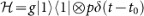between the qubit system and a measuring device and a follow-up post-selection of the qubit system onto the state. The weak value isand the average shift of the pointer momentum is given by. Although the phase ϑ is small, the average shift of the pointer momentum is not necessarily small and this has certain advantages over the quantum-entanglement based scheme in Ramsey interferometry40, which relies on the use of GHZ states of many qubits, which are an expensive resource.

## Discussion

In this article, we have proposed several schemes for state tomography via weak measurements. An advantage of our schemes is that we need to measure fewer observables compared with the standard method of state tomography based on ideal measurements. For example, a simple standard state tomography requires ideal measurements in at least d + 1 different bases; therefore, one needs to use at least d + 1 different experimental setups. Here, our tomography strategy requires weak interaction in one basis ({|ai〉}) and post-selection in another basis ({|bj〉}) and the same interaction Hamiltonian is used for all data collection.

Compared with the scheme in (Ref. 4), our strategy applies not only to pure states but also to mixed states. Our strategy is more efficient because we retain all of the data and thus reduces the number of experimental runs needed. Compared with the schemes in (Ref. 34) in which a density matrix is directly measured via sequential weak measurements of pairs or triple products of observables, our schemes are based on a single-time weak measurement followed by a strong complete projective measurement. Our interaction Hamiltonian in Eq. (19) is straightforward and differs significantly from those in the schemes of (Ref. 34). In the previous schemes4,34, the observables Ai = |ai〉 〈ai| require separate weak measurements at each time for a particular i. In our schemes, the set of observables {Ai} can be measured simultaneously via the interaction introduced by the simple Hamiltonian in Eq. (19).

Interestingly, any matrix element of a density state is directly accessible from a suitable weak measurement. Ideal measurements give probabilities and expectation values, which are only linear combinations of the matrix elements of the density operator. Based on all of these facts, it is natural to draw the conclusion: what is and can be really measured in a general (ideal or weak) measurement is the density matrix (with all its elements) and only the density matrix!

## Methods

### An alternative scheme for the tomography of a pure state via the weak measurement of a single observable

In the main text, we see that it is sufficient to perform a weak measurement of a single observable for the tomography of a pure-state wave function |ψ〉. This single observable could be chosen alternatively as follows. We introduce a single observable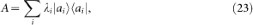where the eigenvalues λi are not degenerated, i.e., λi ≠ λj if ij. We simply perform a weak measurement of the observable A and retain all of the data corresponding to different final states |bj〉. When the final state is |bj〉, the weak value of A is given by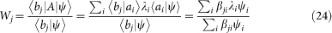From Eq. (24) we have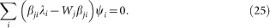We define a unitary matrix β with the matrix elements given by the coefficients βji and introduce the diagonal matrices λ = diag0, λ1, ···, λd−1}, w = diag{w0, w1, ···, wd−1} and the vector, where T denotes the transpose. Then, Eq. (25) can be written as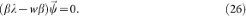With the notation M = βλ − , we have. Thus, the state tomography in this case is to find the vectorcorresponding to the zero eigenvalue of M. The matrix M depends on w, which is determined by the weak values that are directly read out from the experiments. Due to noise and imperfections in the experiments, matrix M may not have a zero eigenvalue. Instead, multiplyingby M from the left, we have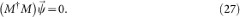Therefore, the vectoris the eigenvector in the kernel space of the non-negative matrix MM. The vectoris uniquely determined if MM has a one-dimensional kernel space. It is not determined and another set of measurements must be performed if MM has a kernel space of more than one dimension. However, it is also possible that the matrix MM determined from the experiment may not have a kernel space. In such a case, we choose the most likely one, i.e., we choose the vectoras the eigenvector corresponding to the smallest eigenvalue of MM. The value of the smallest eigenvalue of MM indicates the amount of noise and imperfection in the experiment.

### Derivation of the position shift and the momentum shift

Here, we derive the position shift and the momentum shift in (20) and (21), respectively. The time evolution operator corresponding to the interaction Hamiltonian in (19) is given by. If the system is successfully post-selected by, which occurs with a probability (to the first order of gi) of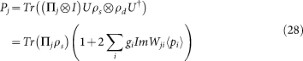the final (unnormalised) state of the pointers is given by (to the first order of gi)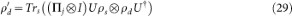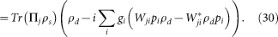Here, Trs (Tr) denotes the trace over the system (whole) Hilbert space. The average position shift of the ith pointer, conditional on the successful post-selction by, is given by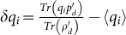. A straightforward calculation to the first order of gi gives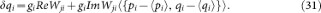When the initial states of the pointers are well-behaved states, such as Gaussian states, the second term on the right-hand side of (31) vanishes and we immediately have (20). Similarly the average momentum shift of the ith pointer, conditional on the successful post-selection by, is given by, which yields (21) to the first order of gi.

## References

1. Bohr, N. The quantum postulate and the recent developments of atomic theory. Naturwissenschaften 16, 245–257 (1928).

2. Aharonov, Y., Albert, D. Z. & Vaidman, L. How the result of a measurement of a component of the spin of a spin-1/2 particle can turn out to be 100. Phys. Rev. Lett. 60, 1351–1354 (1988).

3. Kocsis, S., et al. Observing the average trajectories of single photons in a two-slit interferometer. Science 332, 1170–1173 (2011).

4. Lundeen, J. S., Sutherland, B., Patel, A., Stewart, C. & Bamber, C. Direct measurement of the quantum wavefunction. Nature 474, 188–191 (2011).

5. Ritchie, N. W. M., Story, J. G. & Hulet, R. G. Realization of a measurement of a weak value. Phys. Rev. Lett. 66, 1107–1110 (1991).

6. Pryde, G. J., O'Brien, J. L., White, A. G., Ralph, T. C. & Wiseman, H. M. Measurement of quantum weak values of photon polarization. Phys. Rev. Lett. 94, 220405 (2005).

7. Hosten, O. & Kwiat, P. Observation of the spin Hall effect of light via weak measurements. Science 319, 787 (2008).

8. Aharonov, Y., Botero, A., Popescu, S., Reznik, B. & Tollaksen, J. Revisiting Hardy's paradox: counterfactual statements, real measurements, entanglement and weak values. Phys. Lett. A 301, 130 (2002).

9. Wiseman, H. M. Grounding Bohmian mechanics in weak values and bayesianism. New J. Phys. 9, 165 (2007).

10. Mir, R., Lundeen, J. S., Mitchell, M. W., Steinberg, A. M., Garretson, J. L. & Wiseman, H. M. A double-slit ‘which way’ experiment on the complementarity-uncertainty debate. New J. Phys. 9, 287 (2007).

11. Williams, N. S. & Jordan, A. N. Weak values and the Leggett-Garg inequality in solid-state qubits. Phys. Rev. Lett. 100, 026804 (2008).

12. Johansen, L. M. Weak Measurements with Arbitrary Probe States. Phys. Rev. Lett. 93, 120402 (2004).

13. Hosten, O. & Kwiat, P. Observation of the spin hall effect of light via weak measurements. Science 319, 787 (2008).

14. Dixon, P. B., Starling, D. J., Jordan, A. N. & Howell, J. C. Ultrasensitive beam deflection measurement via interferometric weak value amplification. Phys. Rev. Lett. 102, 173601 (2009).

15. Starling, D. J., Dixon, P. B., Jordan, A. N. & Howell, J. C. Optimizing the signal-to-noise ratio of a beam-deflection measurement with interferometric weak values. Phys. Rev. A 80, 041803(R) (2009).

16. Brunner, N. & Simon, C. Measuring Small Longitudinal Phase Shifts: Weak Measurements or Standard Interferometry? Phys. Rev. Lett. 105, 010405 (2010).

17. Zilberberg, O., Romito, A. & Gefen, Y. Charge sensing amplification via weak values measurement. Phys. Rev. Lett. 106, 080405 (2011).

18. Feizpour, A., Xing, X. & Steinberg, A. M. Amplifying single-photon nonlinearity using weak measurements. Phys. Rev. Lett. 107, 133603 (2011).

19. Agarwal, G. S. & Pathak, P. K. Realization of quantum-mechanical weak values of observables using entangled photons. Phys. Rev. A 75, 032108 (2007).

20. Wu, S. & Żukowski, M. Feasible optical weak measurements of complementary observables via a single Hamiltonian. Phys. Rev. Lett. 108, 080403 (2012).

21. Jozsa, R. Complex weak values in quantum measurement. Phys. Rev. A 76, 044103 (2007).

22. Duck, I. M., Stevenson, P. M. & Sudarshan, E. C. G. The sense in which a ”weak measurement” of a spin-1/2 particle's spin component yields a value 100. Phys. Rev. D 40, 2112 (1989).

23. Starling, D. J., Dixon, P. B., Williams, N. S., Jordan, A. N. & Howell, J. C. Continuous phase amplification with a Sagnac interferometer. Phys. Rev. A 82, 011802(R) (2010).

24. Geszti, T. Postselected weak measurement beyond the weak value. Phys. Rev. A 81, 044102 (2010).

25. Wiseman, H. M. Weak values, quantum trajectories and the cavity-QED experiment on wave-particle correlation. Phys. Rev. A 65, 032111 (2002).

26. Wu, S. & Mølmer, K. Weak measurements with a qubit meter. Phys. Lett. A 374, 34 (2009).

27. Wu, S. & Li Y. Weak measurements beyond the Aharonov-Albert-Vaidman formalism. Phys. Rev. A 83, 052106 (2011).

28. Zhu, X. et al. Quantum measurements with preselection and postselection. Phys. Rev. A 84, 052111 (2011).

29. Nakamura, K., Nishizawa, A. & Fujimoto, M.-K. Evaluation of weak measurements to all orders. Phys. Rev. A 85, 012113 (2012).

30. Pang, S., Wu, S. & Chen, Z.-B. Weak measurement with orthogonal preselection and postselection. Phys. Rev. A 86, 022112 (2012).

31. Kofman, A. G., Ashhab, S. & Nori, F. Nonperturbative theory of weak pre- and post-selected measurements. Phys. Rep. 520, 43–133 (2012).

32. Dressel, J., Agarwal, S. & Jordan, A. N. Contextual values of observables in quantum measurements. Phys. Rev. Lett. 104, 240401 (2010).

33. Kedem, Y. & Vaidman, L. Modular values and weak values of quantum observables. Phys. Rev. Lett. 105, 230401 (2010).

34. Lundeen, J. S. & Bamber, C. Procedure for direct measurement of general quantum states using weak measurement. Phys. Rev. Lett. 108, 070402 (2012).

35. Johansen, L. M. Quantum theory of successive projective measurements. Phys. Rev. A 76, 012119 (2007).

36. Hofmann, H. F. Complex joint probabilities as expressions of reversible transformations in quantum mechanics. New J. Phys. 14, 043031 (2012).

37. Resch, K. J. Amplifying a tiny optical effect. Science 319, 733 (2008).

38. Aharonov, Y., Popescu, S. & Tollaksen, J. A time-symmetric formulation of quantum mechanics. Phys. Today 63, 27 (2010).

39. Salvail, J. Z. et al. Full characterisation of polarisation states of light via direct measurement. ArXiv:1206.2618 [quant-ph].

40. Giovannetti, V., Lloyd, S. & Maccone, L. Advances in quantum metrology. Nature Photonics 5, 222–229 (2011).

## Acknowledgements

The author likes to thank Marek Żukowski for valuable discussions and wishes to acknowledge support from the National Natural Science Foundation of China (Grants No. 11075148 and No. 11275181), the Chinese Academy of Sciences and the National Fundamental Research Program.

Authors

## Ethics declarations

### Competing interests

The author declares no competing financial interests.

## Rights and permissions

Reprints and Permissions

Wu, S. State tomography via weak measurements. Sci Rep 3, 1193 (2013). https://doi.org/10.1038/srep01193

• Accepted:

• Published:

• ### Estimating the coupling strength of a Hamiltonian with four different measurement strategies

• Yawen Jiang
• , Jia-Yi Lin
• , Zhenkai Wang
• , Han Zhang
•  & Shengjun Wu

Modern Physics Letters A (2020)

• ### Measurement of the wavefunction for a biphoton state with homodyne detection using least squares estimation

• Yashuai Han
• , Daohua Wu
• , Katsuyuki Kasai
• , Lirong Wang
• , Masayoshi Watanabe
•  & Yun Zhang

Journal of Optics (2020)

• ### Simulating the effect of weak measurements by a phase damping channel and determining different measures of bipartite correlations in nuclear magnetic resonance

• Akanksha Gautam
• , Amandeep Singh
• , Kavita Dorai
•  & Arvind

Physics Letters A (2020)

• ### Direct measurement methods of density matrix of an entangled quantum state

• Yusuf Turek

Journal of Physics Communications (2020)

• ### Experimental random-party entanglement distillation via weak measurement

• Zheng-Da Li
• , Xiao Yuan
• , Xu-Fei Yin
• , Li-Zheng Liu
• , Rui Zhang
• , Yue-Yang Fei
• , Li Li
• , Nai-Le Liu
• , Xiongfeng Ma
• , He Lu
• , Yu-Ao Chen
•  & Jian-Wei Pan

Physical Review Research (2020)